Next: Vector addition Up: Motion in 3 dimensions Previous: Cartesian coordinates

## Vector displacement

Consider the motion of a body moving in 3 dimensions. The body's instantaneous position is most conveniently specified by giving its displacement from the origin of our coordinate system. Note, however, that in 3 dimensions such a displacement possesses both magnitude and direction. In other words, we not only have to specify how far the body is situated from the origin, we also have to specify in which direction it lies. A quantity which possesses both magnitude and direction is termed a vector. By contrast, a quantity which possesses only magnitude is termed a scalar. Mass and time are scalar quantities. However, in general, displacement is a vector.

The vector displacement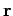of some pointfrom the origincan be visualized as an arrow running from pointto point. See Fig. 11. Note that in typeset documents vector quantities are conventionally written in a bold-faced font (e.g.,) to distinguish them from scalar quantities. In free-hand notation, vectors are usually under-lined (e.g.,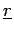).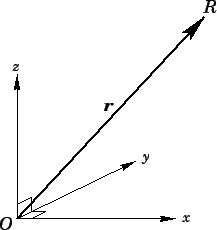The vector displacementcan also be specified in terms of its coordinates: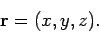(30)

The above expression is interpreted as follows: in order to get from pointto point, first movemeters along the-axis (perpendicular to both the- and-axes), then movemeters along the-axis (perpendicular to both the- and-axes), finally movemeters along the-axis (perpendicular to both the- and-axes). Note that a positivevalue is interpreted as an instruction to movemeters along the-axis in the direction of increasing, whereas a negativevalue is interpreted as an instruction to move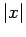meters along the-axis in the opposite direction, and so on.Next: Vector addition Up: Motion in 3 dimensions Previous: Cartesian coordinates
Richard Fitzpatrick 2006-02-02